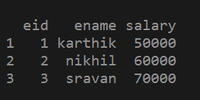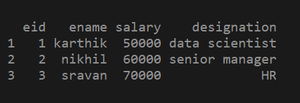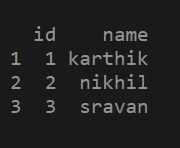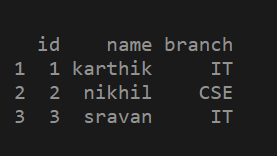Related Articles

# How to add column to dataframe in R ?

• Last Updated : 26 Mar, 2021

In this article, we are going to see how to add columns to dataframe in R. First, let’s create a sample dataframe.

## Adding Column to the DataFrame

We can add a column to a data frame using \$ symbol.

syntax: dataframe_name \$ column_name = c( value 1,value 2 . . . , value n)

Here c() function is a vector holds values .we can pass any type of data with similar type.

Steps for adding a column to a dataframe.

1. Create a data frame.
2. Use the \$ symbol as shown in the above syntax to add a column to a data frame.
3. Print the updated data frame to see the changes.

Example 1: Creating a data frame.

## R

 `# Creating a data frame with eid , ename,salary as columns``df2 = ``data.frame``(eid = ``c``(1, 2, 3),``                 ``ename = ``c``(``"karthik"``, ``"nikhil"``, ``"sravan"``),``                 ``salary = ``c``(50000, 60000, 70000))`` ` `# printing the data frame``print``(df2)`Adding a column using \$ symbol:

## R

 `# creating a data frame``df2 = ``data.frame``(eid = ``c``(1, 2, 3),``                 ``ename = ``c``(``"karthik"``, ``"nikhil"``, ``"sravan"``),``                 ``salary = ``c``(50000, 60000, 70000))`` ` `# adding a new column to the data frame using \$ symbol``df2\$designation = ``c``(``"data scientist"``, ``"senior manager"``, ``"HR"``) `` ` `# printing the updated data frame``print``(df2)`Example 2: Creating a data frame

## R

 `# creating a data frame using vectors``df1 = ``data.frame``(id = ``c``(1, 2 ,3),``                 ``name = ``c``(``"karthik"``, ``"nikhil"``, ``"sravan"``))`` ` `# printing the data frame``print``(df1)`Adding a column to the data frame

## R

 `# creating a data frame``df1 = ``data.frame``(id = ``c``(1, 2, 3),``                 ``name = ``c``(``"karthik"``, ``"nikhil"``, ``"sravan"``))``# adding a new column to the data frame using \$ symbol``df1\$branch = ``c``(``"IT"``, ``"CSE"``, ``"IT"``)`` ` `# printing the updated data frame``print``(df1)`My Personal Notes arrow_drop_up